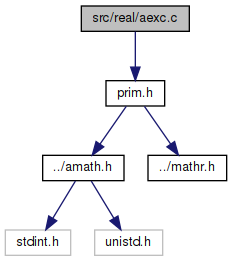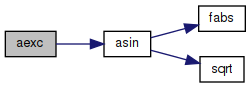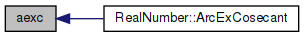amath  1.8.5 Simple command line calculator
aexc.c File Reference
`#include "prim.h"`
Include dependency graph for aexc.c:Go to the source code of this file.

## Functions

double aexc (double x)
Inverse excosecant function. More...

## ◆ aexc()

 double aexc ( double x )

Inverse excosecant function.

```Method
aexcsc(x) = arccsc(x+1)
= arcsin(1/(x+1))
```

Definition at line 46 of file aexc.c.

References asin().

Referenced by RealNumber::ArcExCosecant().

47 {
48  double a, b, c;
49
50  if (x > -2.0 && x < 0.0)
51  return NAN;
52
53  a = x + 1;
54  b = 1 / a;
55  c = asin(b);
56  return c;
57 }
double asin(double x)
Inverse sine function.
Definition: asin.c:100
#define NAN
Definition: mathr.h:53
Here is the call graph for this function:Here is the caller graph for this function: